## Example Questions

### Example Question #1 : How To Find The Slope Of Parallel Lines

Quantity A: The slope of a line parallel to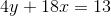Quantity B: The slope of a line perpendicular to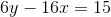Which of the following is true?

The two quantities are equal.

The relationship between the quantities cannot be determined from the information provided.

Quantity B is larger.

Quantity A is larger.

Quantity B is larger.

Explanation:

For a question like this, it is easiest to start by putting each equation into the slope-intercept form.  This is, whereis the slope of the line.

Quantity A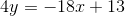Divide both sides by: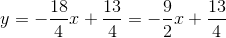So, Quantity A is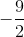.  Parallel lines have equal slopes.

Quantity B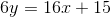Divide both sides by: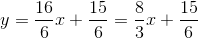Now, the perpendicular of a line has a slope that is opposite and reciprocal.  Therefore, the slope for Quantity B is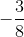.  This is a smaller negative number than the value for Quantity A. Therefore, absolutely speaking, it is a larger value. Therefore, Quantity B is larger.

### Example Question #2 : How To Find The Slope Of Parallel Lines

Quantity A: The slope of the line parallel to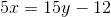Quantity B: The slope of the line parallel to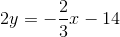Which of the following is true?

Quantity B is larger.

The relationship between the quantities cannot be determined for the information given.

The two quantities are equal.

Quantity A is larger.

Quantity A is larger.

Explanation:

To begin, put each equation into slope-intercept form, which is.  The value foris the slope of the line. The lines that are parallel to each of our given lines will have equal slopes to their respective lines. (Parallel lines have equal slopes, after all.)

Quantity A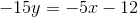Divide both sides by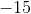: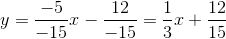Therefore, the slope of the line parallel to this one is.

Quantity BDivide both sides by: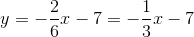Therefore, the slope of the line parallel to this one is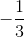.  Therefore, Quantity A is larger.

### Example Question #31 : Algebra

What is the slope of a line parallel to the line: -15x + 5y = 30 ?

3

-15

1/3

30

3

Explanation:

First, put the equation in slope-intercept form: y = 3x + 6. From there we can see the slope of this line is 3 and since the slope of any line parallel to another line is the same, the slope will also be 3.

### Example Question #4 : How To Find The Slope Of Parallel Lines

What is the slope of any line parallel to –6x + 5y = 12?

5/6

6

12

12/5

6/5

6/5

Explanation:

This problem requires an understanding of the makeup of an equation of a line.  This problem gives an equation of a line in y = mx + b form, but we will need to algebraically manipulate the equation to determine its slope.  Once we have determined the slope of the line given we can determine the slope of any line parallel to it, becasue parallel lines have identical slopes.  By dividing both sides of the equation by 5, we are able to obtain an equation for this line that is in a more recognizable y = mx + b form. The equation of the line then becomes y = 6/5x + 12/5, we can see that the slope of this line is 6/5.

### Example Question #11 : Coordinate Geometry

What is the slope of a line that is parallel to the line 11x + 4y - 2 = 9 – 4x  ?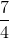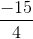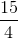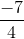Explanation:

We rearrange the line to express it in slope intercept form.

Any line parallel to this original line will have the same slope.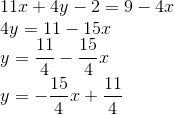### Example Question #21 : Parallel Lines

In the standard (x, y) coordinate plane, what is the slope of a line parallel to the line with equation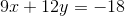?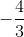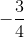Explanation:

Parallel lines will have equal slopes. To solve, we simply need to rearrange the given equation into slope-intercept form to find its slope.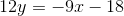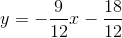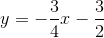The slope of the given line is. Any lines that run parallel to the given line will also have a slope of.

### Example Question #7 : How To Find The Slope Of Parallel Lines

What is the slope of a line that is parallel to the line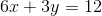?Explanation:

Parallel lines have the same slope. The question requires you to find the slope of the given function. The best way to do this is to put the equation in slope-intercept form (y = mx + b) by solving for y.

First subtract 6x on both sides to get 3y = –6x + 12.

Then divide each term by 3 to get y = –2x + 4.

In the form y = mx + b, m represents the slope. So the coefficient of the x term is the slope, and –2 is the correct answer.

### Example Question #8 : How To Find The Slope Of Parallel Lines

A certain line has points at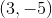and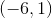. Which of the following lines is parallel to the so-called "certain" line?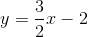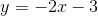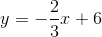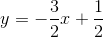Explanation:

To begin, we must first solve the for the slope of the original line. Use the formula for slope to do this: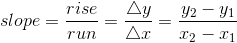Use the two points we were given: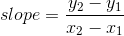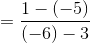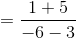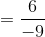Reduce the fraction: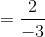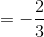We are looking for any answer choice with a slope of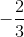. The answer is.

Tired of practice problems?

Try live online GRE Math prep today.